# Class 12 Maths NCERT Solutions for Chapter 11 Three Dimensional Geometry Miscellaneous Exercise### Three Dimensional Geometry Miscellaneous Exercise Solutions

1. Show that the line joining the origin to the point (2, 1, 1) is perpendicular to the line determined by the points (3, 5, – 1), (4, 3, – 1).

Solution

Let OA be the line joining the origin, O (0, 0, 0), and the point, A (2, 1, 1).
Also, let BC be the line joining the points, B (3, 5, −1) and C (4, 3, −1).
The direction ratios of OA are 2, 1, and 1 and of BC are (4 − 3) = 1, (3 − 5) = −2, and (−1 + 1) = 0
OA is perpendicular to BC, if a1a2 + b1b2 + c1c2 = 0
∴ a1a2 + b1b2 + c1c2 = 2×1 + 1 (−2) + 1×0 = 2 − 2 = 0
Thus, OA is perpendicular to BC.

2. If l1m1n1 and l2m2n2 are the direction cosines of two mutually perpendicular lines, show that the direction cosines of the line perpendicular to both of these are m1n2 − m2n1n1l2 − n2l1l1m2 ­− l2m1

Solution

It is given that l1m1n1 and l2m2n2 are the direction cosines of two mutually perpendicular lines. Therefore,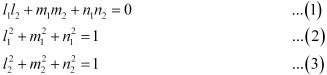Let l, m, n be the direction cosines of the line which is perpendicular to the line with direction cosines  l1m1n1 and l2m2n2 .
∴ ll1, mm1, nn1 = 0
ll2, mm2, nn2  = 0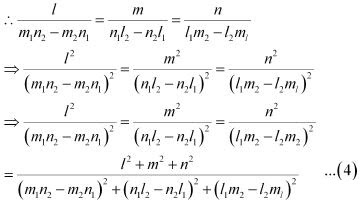l, m, n are the direction cosines of the line.
Therefore, l2 + m2 + n2 = 1 …(5)
It is known that,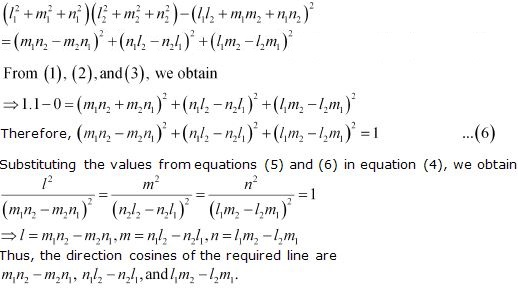3. Find the angle between the lines whose direction ratios are aband bccaab.

Solution

The angle Q between the lines with direction cosines, a, b, c and b-c, c-a, a-b is given by,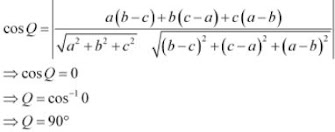Thus, the angle between the lines is 90°.

4. Find the equation of a line parallel to x-axis and passing through the origin.

Solution

The line parallel to x-­­axis and passing through the origin is x-axis itself.
Let A be a point on x-axis. Therefore, the coordinates of A are given by (a, 0, 0), where a ∈ R.
Direction ratios of OA are (a − 0) = a, 0, 0
The equation of OA is given by,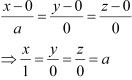Thus, the equation of line parallel to x - axis and passing through origin is
x/1 = y/0 = z/0

5. If the coordinates of the points A, B, C, D be (1, 2, 3), (4, 5, 7), (­−4, 3, −6) and (2, 9, 2) respectively, then find the angle between the lines AB and CD.

Solution

The coordinates of A, B, C, and D are (1, 2, 3), (4, 5, 7), (­−4, 3, −6), and (2, 9, 2) respectively.
The direction ratios of AB are (4 − 1) = 3, (5 − 2) = 3, and (7 − 3) = 4
The direction ratios of CD are (2 −(− 4)) = 6, (9 − 3) = 6, and (2 −(−6)) = 8
It can be seen that,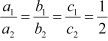Therefore, AB is parallel to CD.
Thus, the angle between AB and CD is either 0° or 180°.

6. If the lines  (x - 1)/-3 = (y - 2)/2k = (z - 3)/2 and (x -1)/3k = (y - 1)/1 = (z - 6)/-5 are perpendicular, find the value of k.

Solution

The direction of ratios of the lines, (x - 1)/-3 = (y - 2)/2k = (z - 3)/2 and (x -1)/3k = (y - 1)/1 = (z - 6)/-5  are -3, 2k, 2 and 3k, 1, -5 respectively.
It is known that two lines with direction ratios, a1 , b1 , c1 ,and a2 , b2 , c2 are perpendicular , if a1a2 + b1b2 + c1c2  = 0
∴ -3(3k) + 2k × 1 + 2(-5) = 0
⇒ -9k + 2k - 10 = 0
⇒ 7k = -10
⇒ k = -10/7
Therefore, for k = -10/7 , the given lines are perpendicular to each other.

7. Find the vector equation of the plane passing through (1, 2, 3) and perpendicular to the plane    r⃗ .(i ^+ 2j ^- 5k ^)+9 = 0

Solution

The position vector of the point (1, 2, 3) is r1 = i + 2j + 3k ^ .
The direction ratios of the normal to the plane,  r.(i + 2j - 5k ^) + 9 = 0 are 1, 2, and -5 and the normal vector is n = (i + 2j - 5k ^
The equation of a line passing through a point and perpendicular to the given plane is given by,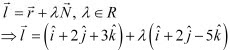8. Find the equation of the plane passing through (a, b, c) and parallel to the plane r. (i ^+ j ^+ k ^) =2

Solution

Any plane parallel to the plane,  r1⃗ .(i ^+ j + k ^) = 2 , is of the form
r⃗ .(i + j + k ^) = λ  ...(1)
The plane passes through the point (a, b, c). Therefore, the position vector  r⃗  of this point
r⃗  = ai + bj + ck ^
Therefore, equation (1) becomes
(ai + bj + ck ^).(i + j + k ^)  = λ
⇒ a + b + c = λ
Substituting λ = a + b + c in equation (1), we obtain
r⃗ .(i + j + k ^) = a + b + c ...(2)
This is the vector equation of the required plane.
Substituting r = xi + yj + zk ^ in equation (2), we obtain
(xi + yj + zk ^).(i + j + k ^) = a + b + c
⇒ x + y + z = a + b+ c

9. Find the shortest distance between lines r⃗  = 6i ^+ 2j ^+ 2k ^+ λ(i ^- 2j ^+ 2k ^) and r = -4i ^- k ^+ μ(3i ^- 2j ^- 2k ^).

Solution

The given lines are

r = 6i + 2j + 2k ^ + λ(i ^- 2j ^+ 2k ^) ...(1)
r⃗ = -4i - k ^+ μ(3i - 2j - 2k ^) ...(2)
It is known that the shortest distance between two lines,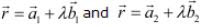, is given by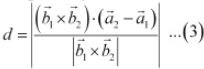Comparing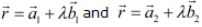to equations (1) and (2), we obtain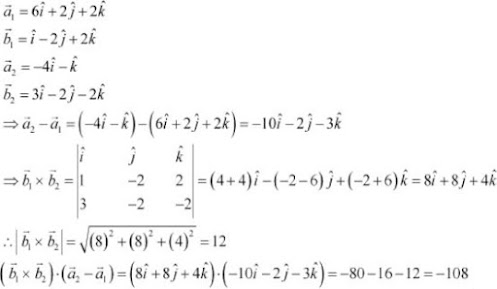Substituting all the values in equation (1), we obtain
d= |-108/12| = 9
Therefore, the shortest distance between the two given lines is 9 units.

10. Find the coordinates of the point where the line through (5, 1, 6) and (3, 4, 1) crosses the YZ-plane

Solution

It is known that the equation of the line passing through the points, (x1y1z1) and (x2y2z2), is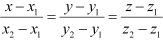The line passing through the points, (5, 1, 6) and (3, 4, 1), is given by,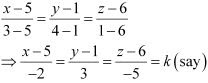⇒ x = 5 - 2k, y = 3k + 1, z = 6 - 5k
Any point on the line is of the form (5 - 2k, 3k + 1, 6 - 5k).
The equation of YZ - plane is x = 0
Since the line passes through YZ - plane,
5 -2k = 0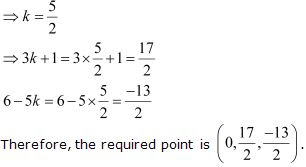11. Find the coordinates of the point where the line through (5, 1, 6) and (3, 4, 1) crosses the ZX − plane.

Solution

It is known that the equation of the line passing through the points, (x1y1z1) and (x2y2z2), isThe line passing through the points, (5, 1, 6) and (3, 4, 1), is given by,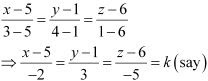⇒ x = 5 - 2k, y = 3k + 1, z = 6 - 5k
Any point on the line is of the form (5 - 2k, 3k + 1, 6 - 5k).
Since the line passes through ZX - plane,
3k + 1 = 0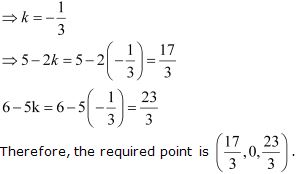12. Find the coordinates of the point where the line through (3, ­−4, −5) and (2, −3, 1) crosses the plane 2x + z = 7).

Solution

It is known that the equation of the line through the points, (x1y1z1) and (x2y2z2), isSince the line passes through the points, (3, -4, -5) and (2, -3, 1) its equation is given by,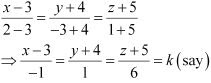⇒ x = 3 - k, y = k - 4, z = 6k - 5
Therefore, any point on the line is of the form (3 - k, k - 4, 6k - 5).
This point lies on the plane, 2x + y + z = 7
Therefore, 2(3 - k) + (k - 4) + (6x - 5) = 7
⇒ 5k - 3 = 7
⇒ k = 2
Hence, the coordinates of the required point are (3, -2, 2 - 4, 6×2 - 5) i.e., (1, -2, 7).

13. Find the equation of the plane passing through the point (−1, 3, 2) and perpendicular to each of the planes x + 2+ 3z = 5 and 3x + 3z = 0.

Solution

The equation of the plane passing through the point (−1, 3, 2) is
(x + 1) + b (y − 3) + c (z − 2) = 0  …(1)
where, abc are the direction ratios of normal to the plane.
It is known that two planes,  a1x + b1y + c1z + d1 = 0 and a2x + b2y + c2z + d2 = 0 perpendicular , if  a1a2 + b1b2 + c1c2 = 0
Plane (1) is perpendicular to the plane, x + 2y + 3z = 5
∴ a.1 + b.2 +  c.3 = 0
⇒ a + 2b + 3c = 0  ...(2)
Also, plane (1) is perpendicular to the plane, 3x + 3y + z = 0
∴ a.3 + b.3 + c.1 = 0
⇒ 3a + 3b + c = 0  ...(3)
From equations (2) and (3), we obtain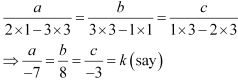⇒ a = -7k , b = 8k, c = -3k
Substituting the values of a, b, and c in equation (1), we obtain
-7k(x + 1) + 8k(y - 3) - 3k(z - 2) = 0
⇒ (-7x - 7) + (8y - 24) - 3z + 6 = 0
⇒ -7x + 8y - 3z - 25 = 0
⇒ 7x - 8y + 3z + 25 = 0
This is the required equation of the plane.

14. If the points (1, 1, p) and (−3, 0, 1) be equidistant from the plane r⃗ .(3i ^+ 4j ^- 12k ^) + 13 = 0 then find the value of p.

Solution

The position vector through the point (1, 1, p) is a1⃗  = i +  j +  pk ^  .
Similarly, the position vector through the point (-3, 0, 1) is
a2⃗ = -4i ^+ k ^
The equation of the given plane is  r.(3i + 4j - 12k ^) + 13 = 0
It is known that the perpendicular distance between a point whose position vector is  a⃗  and the plane,

r.N⃗  = d, is given by ,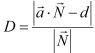Here, N⃗  = 3i + 4j - 12k ^ and d = -13
Therefore, the distance between the point (1, 1, p) and the given plane is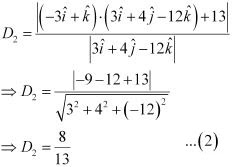It is given that the distance between the required plane and the points, (1, 1, p) and (-3, 0, 1), is equal.
∴ D1 = D2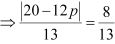⇒ 20 - 12p = 8 or -(20 -12p) = 8
⇒ 12p = 12 or 12p = 28
⇒ p = 1 or p = 7/3

15. Find the equation of the plane passing through the line of intersection of the planes r.(i ^+ j ^+ k ^) = 1 and r.(2i ^+ 3j ^- k ^) + 4 = 0 and parallel to x-axis .

Solution

The given planes are  r .(i + j + k ^) = 1
⇒ r⃗ .(i + j + k ^) -1 = 0

r.(2i + 3j - k ^) + 4 = 0
The equation of any plane passing through the line of intersection of these planes is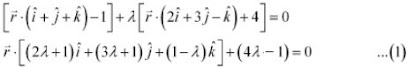Its direction ratios are (2λ + 1), (3λ + 1), and (1 - λ).
The required plane is parallel to x - axis. Therefore, its normal is perpendicular to x - axis.
The direction ratios of x - axis are 1, 0, and 0.
∴ 1.(2λ + 1) + 0(3λ + 1) + 0(1 - λ) = 0
⇒ 2λ + 1 = 0
⇒ λ = -1/2
Substituting λ = -1/2 in equation (1), we obtain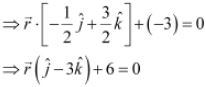Therefore, its Cartesian equation is y - 3z + 6 = 0
This is the equation of the required plane.

16. If O be the origin and the coordinates of P be (1, 2, −3), then find the equation of the plane passing through P and perpendicular to OP.

Solution

The coordinates of the points, O and P, are (0, 0, 0) and (1, 2, −3) respectively.
Therefore, the direction ratios of OP are (1−0) = 1, (2−0) = 2, and (−3 − 0) = −3
It is known that the equation of the plane passing through the point (x1y1z1) is a(x - x1) + b(y - y1) + c(z - z1) = 0 where, a, b, and c are the direction ratios of normal.
Here, the direction ratios of normal are 1, 2, and -3 and the point P is (1, 2, -3).
Thus, the equation of the required plane is
1(x - 1) + 2(y - 2) - 3(z + 3) = 0
⇒ z + 2y - 3z - 14 = 0

17. Find the equation of the plane which contains the line of intersection of the planes r⃗ .(i ^+ 2j ^+ k ^) - 4 = 0, r.(2i + j ^- k ^) + 5 = 0, and which is perpendicular to the plane r.(5i ^+ 3j ^- 6k ^) +8 = 0.

Solution

The equations of the given planes are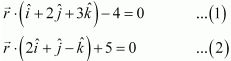The equation of the plane passing through the line intersection of the plane given in equation (1) and equation (2) is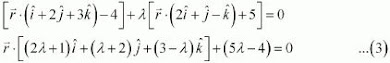The plane in equation (3) is perpendicular to the plane, r.(5i ^+ 3j - 6k ^) + 8 = 0
∴ 5(2λ + 1) + 3(λ+ 2) - 6(3 - λ) = 0
⇒ 19λ - 7 = 0
⇒ λ = 7/19
Substituting λ = 7/19 in equation (3), we obtain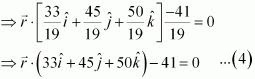This is the vector equation of the required plane.
The Cartesian equation of this plane can be obtained by substituting  r.(xi + yj + zk ^) in equation (3).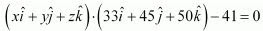⇒ 33x + 45y + 50z - 41 = 0

18. Find the distance of the point (−1, −5, −­10) from the point of intersection of the line r = 2i - j + 2k ^+ λ(3i ^+ 4j ^+ 2k ^) and the plane r.(i ^- j ^+ k ^) = 5.

Solution

The equation of the given line is
r⃗  = 2i - j + 2k + λ( 3i ^+ 4j ^+ 2k ^...(1)
The equation of the given plane is
r⃗ .(i ^- j ^+ k ^) = 5 ...(2)
Substituting the value of  r⃗  from equation (1) in equation (2), we obtain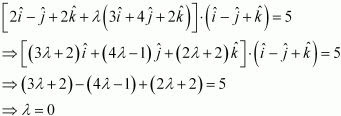Substituting this value in equation (1), we obtain the equation of the line as r = 2i ^- j ^+ 2k ^
This means that the position vector of the point of intersection of the line and the plane is r = 2i ^- j ^+ 2k ^.
This shows that the point of intersection of the given line and plane is given by the coordinates, (2, −1, 2). The point is (−1, −5, −10).
The distance d between the points, (2, −1, 2) and (−1, −5, −10), is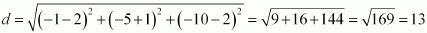19. Find the vector equation of the line passing through (1, 2, 3) and parallel to the planes r⃗ .( i ^- j ^+ 2k ^) = 5 and r⃗ .( 3i ^+ j ^+ k ^) = 6.

Solution

Let the required line be parallel to be parallel to vector b⃗  given by,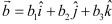The position vector of the point (1, 2, 3) is a = i ^+ 2j ^+3k ^
The equation of line passing through (1, 2, 3) and parallel to b is given by,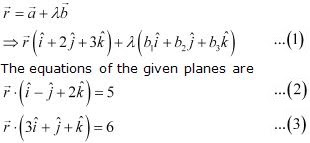The line in equation (1) and plane in equation (2) are parallel. Therefore, the normal to the plane of equation (2) and the given line are perpendicular.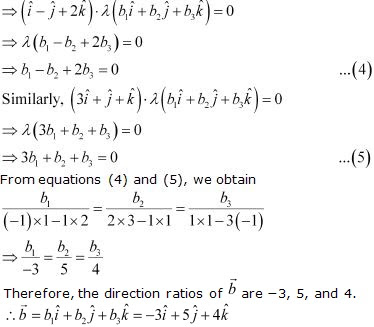Substituting the value of b⃗  in equation (1), we obtain
r = (i ^+2j ^+ 3k ^) + λ(-3i ^+ 5j ^+ 4k ^)
This is the equation of the required line.

20. Find the vector equation of the line passing through the point (1, 2, −4) and perpendicular to the two lines:
(x - 8)/3 = (y + 19)/-16 = (z - 10)/7 and (x - 15)/3 = (y - 29)/8 = (z - 5)/-5

Solution

Let the required line be parallel to the vector b⃗  given by,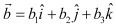The position vector of the point (1, 2, -4) is a = (i + 2j - 4k ^)
The equation of the line passing through (1, 2, -4) and parallel to vector b⃗ is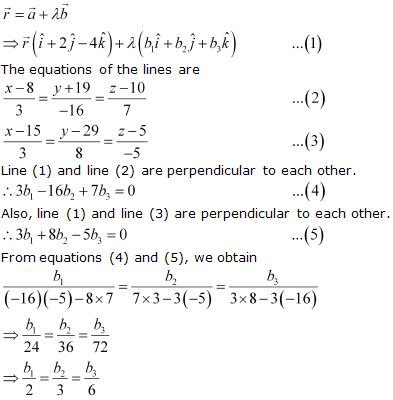∴ Direction ratios of b⃗ are 2, 3 and 6.
∴ b = 2i ^+ 3j ^+ 6k ^
Substituting b⃗ = 2i ^+ 3j ^+ 6k ^ in equation (1), we obtain
r⃗ = (i ^+ 2j ^- 4k ^) + λ(2i ^+ 3j ^+ 6k ^)
This is the equation of the required line.

21. Prove that if a plane has the intercepts abc and is at a distance of P units from the origin, then 1/a2 + 1/b2 + 1/c2 = 1/p2.

Solution

The equation of a plane having intercepts a, b, c with x, y, and z axes respectively is given by,
x/a +y/b +z/c = 1 ...(1)
The distance (p) of the plane from the origin is given by,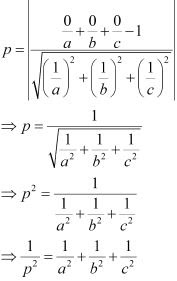22. Distance between the two planes: 2x + 3y + 4z = 4 and 4x + 6y + 8z = 12 is
(A) 2 units
(B) 4 units
(C) 8 units
(D) 2/√29 units

Solution

The equations of the planes are
2x + 3y + 4z = 4  ...(1)
4x + 6y + 8z = 12
⇒ 2x + 3y + 4z = 6  ...(2)
It can be seen that the given planes are parallel .
It is known that the distance between two parallel planes, ax + by + cz = d1 and ax +by +cz = d2, is given by,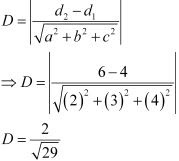Thus, the distance between the lines is 2/√29 units.
Hence, the correct answer is D.

23. The planes: 2− y + 4z = 5 and 5x − 2.5y + 10z = 6 are
(A) Perpendicular
(B) Parallel
(C) intersect y-axis
(C) passes through (0, 0, 5/4)

Solution

The equations of the planes are
2x - y + 4z = 5 ...(1)
5x - 2.5y + 10z = 6  ...(2)
It can be seen that,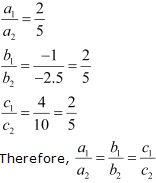Therefore, the given planes are parallel.
Hence, the correct answer is B.